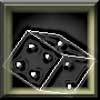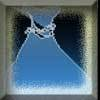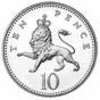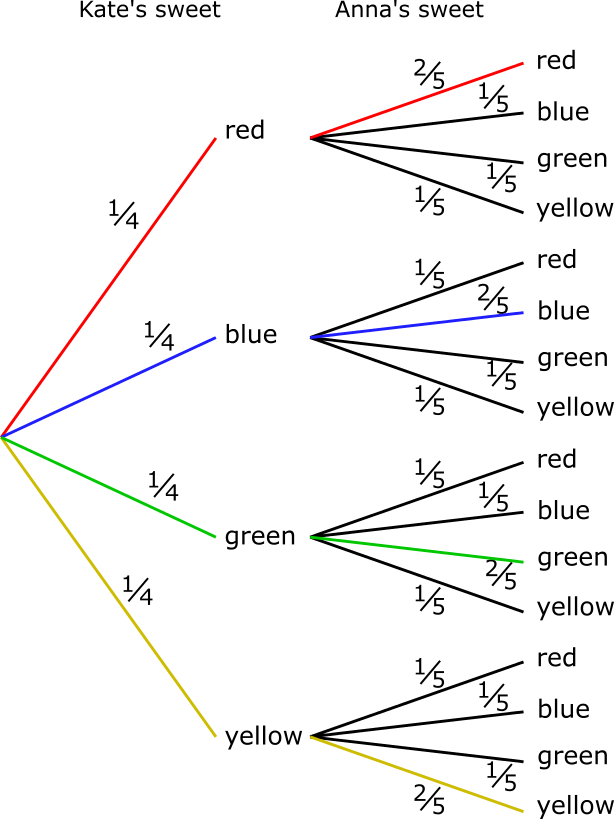You may also likeGambling at Monte Carlo

A man went to Monte Carlo to try and make his fortune. Is his strategy a winning one?Marbles and Bags

Two bags contain different numbers of red and blue marbles. A marble is removed from one of the bags. The marble is blue. What is the probability that it was removed from bag A?Coin Tossing Games

You and I play a game involving successive throws of a fair coin. Suppose I pick HH and you pick TH. The coin is thrown repeatedly until we see either two heads in a row (I win) or a tail followed by a head (you win). What is the probability that you win?

Swapping Sweets

Age 14 to 16 ShortChallenge Level
Using a tree diagram
There are two stages here - the sweet that Kate removes, and the sweet that Anna removes - and so a two-stage tree diagram will be helpful.

When Kate takes a sweet out of her bag, there are 4 to choose from, and they are all different colours - so the probability of choosing each colour is $\frac{1}{4}$.

When Anna takes a sweet out of her bag, there are 5 to choose from. If Kate put a red sweet into Anna's bag, then there are two red sweets, so the probability that Anna chooses a red sweet is $\frac{2}{5}$. There is still only one blue sweet, one green sweet and one yellow sweet, so the probability that she chooses each of the other colours is $\frac{1}{5}$. Similarly, if Kate put a blue sweet into Anna's bag, then the probability that Anna chooses a blue sweet is $\frac{2}{5}$, and the probability that she chooses each of the other colours is $\frac{1}{5}$ - and so on.

This is shown on the tree diagram below.The two bags will each end up with one sweet of each colour if the sweet that Anna puts into Kate's bag is the same colour as the sweet that Kate has put into Anna's bag. Those possibilities are highlighted on the tree diagram below.The probability of going along the red path is $\frac{1}{4}\times\frac{2}{5}=\frac{1}{10}$. The probability of going along each of the other paths is also $\frac{1}{10}$, so the probability that both bags end up containing one sweet of each colour will be $\frac{1}{10}+\frac{1}{10}+\frac{1}{10}+\frac{1}{10}=\frac{4}{10}=\frac{2}{5}$ .

Since there are 5 sweets in the bag and 2 to choose from of the required colour, the probability that Anna chooses one of those is $\frac{2}{5}$.